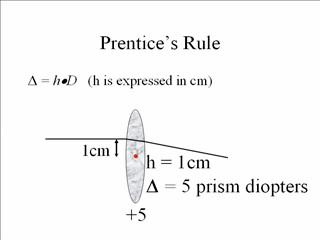# Formulas Flashcards

Set Details Share
created 9 years ago by cdterry916
1,317 views
updated 9 years ago by cdterry916
Page to share:
Embed this setcancel
COPY
code changes based on your size selection
Size:
X

1

Index of Refraction Formula

*Speed of Light in Air

-----------Divided by--------------

Speed of light in Material

*186,000 miles per second

2

Minimum Blank Size Formula (MBS)

Formula = *(GCD minus PD) + ED

*GCD = "Geometric Center Distance" which is calculated like this: (A + DBL)

FYI: PD = pupillary distance. ED is the diagonal measurement of lens in frame. A is width measurement of lens in frame. DBL is distance between lenses a.k.a bridge measurement in frame.

3

Slab Off Formula

• This is a fabrication process in which base-up prism is ground on the lower portion of the lens to redirect light as a patient gazes downward. This technique allows the patient to fuse the images and prevent prism as the eye travels down the vertical meridian of the lens
• Find reading O.C. (distance O.C. minus 10mm)
• Find total power of lenses at 90 degrees
• Use Prentice Rule to find prism difference between right and left lenses

4

Toric Transposition

used to determine lens power in the principal meridians

5

How do you find the total or absolute lens power at 180 degrees or 90 degrees when Rx gives it at 45 degrees or 135 degrees?

Divide cylinder in half and add to sphere

6

How do you determine the lens power 90 degrees away from the cylinder axis?

Algebraically add sphere power to cylinder power

7

Prentice's RuleAmount of induced prism = power of lens x decentration in centimeters

*In the picture, the lens power is +5. The decentration in the picture is 1cm. So, 1cm x power of 5 = 5 prism diopters (the amount of induced prism)

8

Horizontal Decentration Formula

*GCD minus PD divided by 2

*GCD = Geometric Center Distance. PD= Pupillary Distance

9

Definition of Decentration

Put simply, imagine this: Your patient has a specific PD at distance and at near. You measure this by using your pupilometer. The frame that your patient has chosen also has a PD. The frame PD is measured by adding the eye size to the bridge size. Decentration is simply a big word for how far we have to move the optical center in or out, when making the lenses so that when the lens is mounted in the frame, the optical center sits right in front of your patient's pupil. If the optical center does not sit right in front of your patient's pupil, we will create unwanted prism.

10

Vertical Decentration Formula

Seg Height - ("B measurement" / 2)

1. Take the B Measurement and divide it by 2.
2. Take that number and subtract it from the Seg Height